Home > INT3 > Chapter 7 > Lesson 7.2.5 > Problem7-107

7-107.
1. Consider the triangle below. Homework Help ✎

2.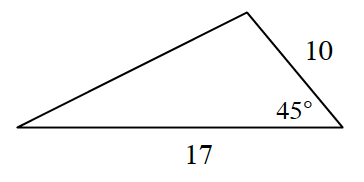1. Solve for the missing side lengths and angles in the triangle.

2. What is the area of the triangle?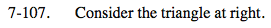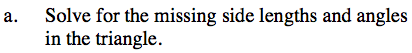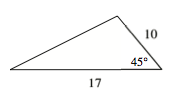This triangle has known values for SAS (side-angle-side) so will need to be solved using the Law of Cosines.
Refer to the Math Notes box in Lesson 7.2.3 if you need help remembering the Law of Cosines.

Solve for the missing side length.

$c^{2}=10^{2}+17^{2}-2(10)(17)\text{ cos }45\degree$

After finding the length of c, the Law of Sines can be used to to determine the missing angle measures. Refer to the Math Notes box in Lesson 7.2.2 for the Law of Sines.

$\frac{\sin A}{10}=\frac{\sin 45 \degree}{c}$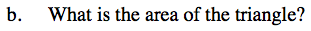Drawing in the height creates a special right triangle.

Using the special relationship, determine the height of the triangle.